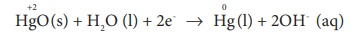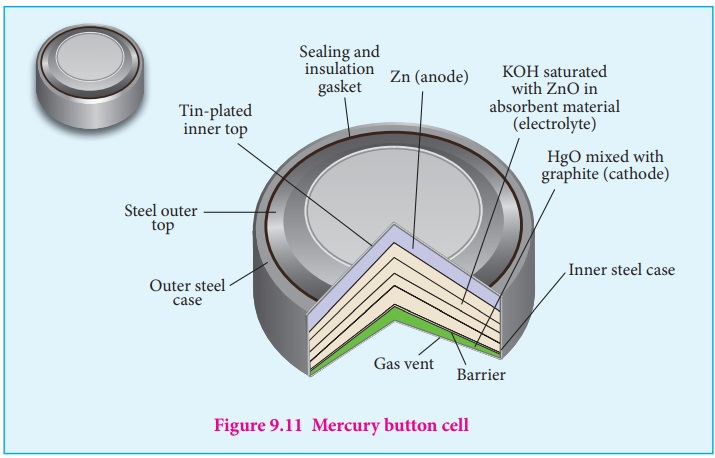Home | | Chemistry 12th Std | Batteries

# Batteries

Batteries are indispensable in the modern electronic world. For example, Li ŌĆō ion batteries are used in cell phones, dry cell in flashlight etcŌĆ”. These batteries are used as a source of direct current at a constant voltage.

Batteries

Batteries are indispensable in the modern electronic world. For example, Li ŌĆō ion batteries are used in cell phones, dry cell in flashlight etcŌĆ”. These batteries are used as a source of direct current at a constant voltage. We can classify them into primary batteries (non ŌĆō rechargeable) and secondary batteries (rechargeable). In this section, we will briefly discuss the electrochemistry of some batteries.

## Leclanche cell

Anode : Zinc container

Cathode : Graphite rod in contact with MnO2

Electrolyte : ammonium chloride and zinc chloride in water

Emf of the cell is about 1.5V

Cell reaction

Oxidation at anode

Zn (s) ŌåÆ Zn2+ (aq)+2e-                     .....(1)

Reduction at cathode

2 NH4+ (aq) + 2e- ŌåÆ 2NH3(aq) + H2 (g)                 .....(2)

The hydrogen gas is oxidised to water by MnO2

H2 (g) + 2 MnO2 (s) ŌåÆ Mn2 O3 (s) + H2O (l)                   .....(3)

Equation (1) + (2) + (3) gives the overall redox reactionZn (s) + 2NH4+ (aq) + 2 MnO2 (s) ŌåÆ Zn2+ (aq) + Mn2 O3(s) + H2O (l) + 2NH3

...... (4)

Ammonia produced at the cathode combines with Zn2+ to form a complex ion [Zn (NH3 )4 ]2+ (aq) . As the reaction proceeds, the concentration of NH4+ will decrease and the aqueous NH3 will increase which lead to the decrease in the emf of cell.

## Mercury button cell

Anode : zinc amalgamated with mercury

Cathode : HgO mixed with graphite

Electrolyte : Paste of KOH and ZnO

Oxidation occurs at anode :Reduction occurs at cathode :Overall reaction : Zn (s) + HgO (s) ŌåÆ ZnO (s) + Hg (l)

Uses : It has higher capacity and longer life. Used in pacemakers, electronic watches, cameras etcŌĆ”## Secondary batteries

We have already learnt that the electrochemical reactions which take place in a galvanic cell may be reversed by applying a potential slightly greater than the emf generated by the cell. This principle is used in secondary batteries to regenerate the original reactants. Let us understand the function of secondary cell by considering the lead storage battery as an example

Cathode : lead plate bearing PbO2

Electrolyte : 38% by mass of H2 SO4 with density 1.2g / mL.

Oxidation occurs at the anode

Pb(s) ŌåÆ Pb2+ (aq)+2e-              .....(1)

The Pb2+ ions combine with SO4-2 to from PbSO4 precipitate.

Pb2+ (aq) + SO4 2ŌłÆ(aq) ŌåÆ PbSO4 (s)                   .....(2)

Reduction occurs at the cathode

PbO2 (s) + 4 H+ (aq) + 2e- ŌåÆ Pb2+ (aq) + 2H2O (l)                 ......(3)

The Pb2+ ions also combine with SO42- ions from sulphuric acid to form precipitate.

PbSO4 Pb2+ (aq) + SO4 2ŌłÆ (aq) ŌåÆ PbSO4 (s)                  .....(4)

The Overall reactions is

Equation (1) + (2) + (3) + (4)

Pb (s) + PbO2 (s) + 4H + (aq) + 2SO4 2ŌłÆ (aq) ŌåÆ 2 PbSO4 (s) + 2H2O (l )

The emf of a single cell is about 2V . Usually six such cells are combined in series to produce 12volt

The emf of the cell depends on the concentration of H2 SO4 . As the cell reaction uses SO42ŌłÆ ions, the concentration H2 SO4 decreases. When the cell potential falls to about 1.8V, the cell has to be recharged.

## Recharge of the cell

As said earlier, a potential greater than 2V is applied across the electrodes, the cell reactions that take place during the discharge process are reversed. During recharge process, the role of anode and cathode is reversed and H2 SO4 is regenerated.

Oxidation occurs at the cathode ( now act as anode)Reduction occurs at the anode (now act as cathode) PbSO4 (s) + 2e- ŌåÆ Pb(s) + SO42-(aq)

Overall reaction

2PbSO4(s) + 2H2O (l ) ŌåÆ Pb (s) + PbO2 (s) + 4H+ (aq) + 2SO42- (aq).Thus, the overall cell reaction is exactly the reverse of the redox reaction which takes place while discharging .

### Uses:

Used in automobiles, trains, inverters etcŌĆ”

## The lithium ŌĆō ion Battery

Anode : Porus graphite

Cathode : transition metal oxide such as CoO2.

Electrolyte : Lithium salt in an organic solvent

At the anode oxidation occurs

Li (s) ŌåÆ Li+ (aq) + e-

At the cathode reduction occurs

Li+ + CoO2 (s) + e- ŌåÆ Li CoO2 (s)

Overall reactions

Li (s) + CoO2 ŌåÆ LiCoO2 (s)Both electrodes allow Li+ ions to move in and out of their structures.

During discharge, the Li+ ions produced at the anode move towards cathode through the non ŌĆō aqueous electrolyte. When a potential greater than the emf produced by the cell is applied across the electrode, the cell reaction is reversed and now the Li+ ions move from cathode to anode where they become embedded on the porous graphite electrode. This is known as intercalation.

### Uses :

Used in cellular phones, laptops, computers, digital cameras, etcŌĆ”

## Fuel cell

The galvanic cell in which the energy of combustion of fuels is directly converted into electrical energy is called the fuel cell. It requires a continuous supply of reactant to keep functioning. The general representation of a fuel cell is follows

Fuel | Electrode | Electrolyte | Electrode | OxidantLet us understand the function of fuel cell by considering hydrogen ŌĆō oxygen fuel cell. In this case, hydrogen act as a fuel and oxygen as an oxidant and the electrolyte is aqueous KOH maintained at 200oC and 20 ŌĆō 40 atm. Porous graphite electrode containing Ni and NiO serves as the inert electrodes.

Hydrogen and oxygen gases are bubbled through the anode and cathode, respectively.

Oxidation occurs at the anode:

2H2 (g ) + 4 OHŌłÆ (aq) ŌåÆ 4 H2O (l) + 4 eŌłÆ

Reduction occurs at the cathode O 2 (g) + 2 H2O (l) + 4eŌłÆ ŌåÆ 4 OHŌłÆ (aq)

The overall reaction is 2H2 (g) + O2 (g) ŌåÆ 2H2O (l)

The above reaction is the same as the hydrogen combustion reaction, however, they do not react directly ie., the oxidation and reduction reactions take place separately at the anode and cathode respectively like H2 - O2 fuel cell. Other fuel cells like propane ŌĆōO2 and methane O2 have also been developed.

Tags : Thermodynamics of cell reactions | Electro Chemistry , 12th Chemistry : UNIT 9 : Electro Chemistry
Study Material, Lecturing Notes, Assignment, Reference, Wiki description explanation, brief detail
12th Chemistry : UNIT 9 : Electro Chemistry : Batteries | Thermodynamics of cell reactions | Electro Chemistry